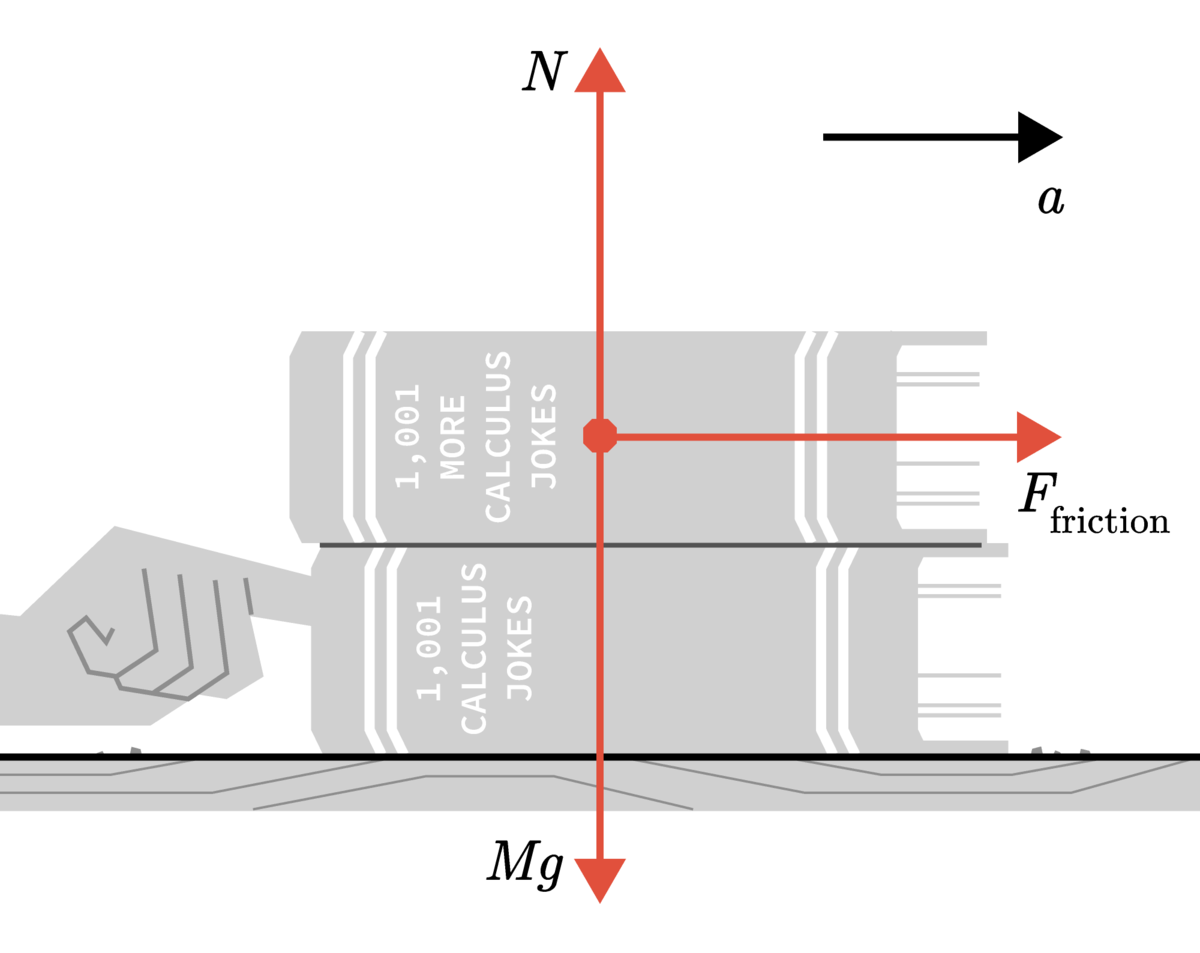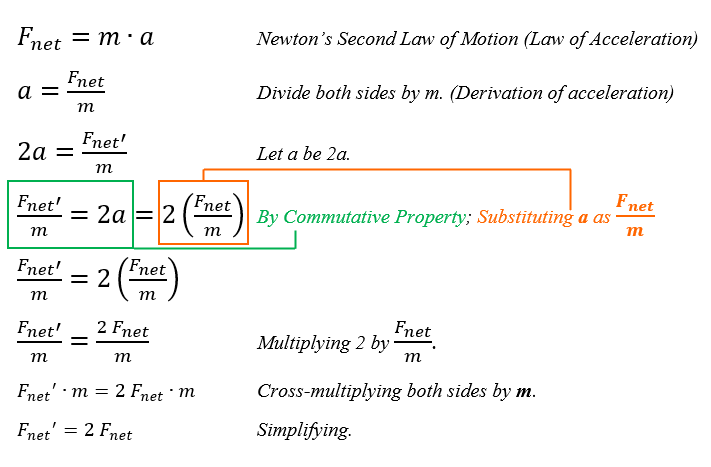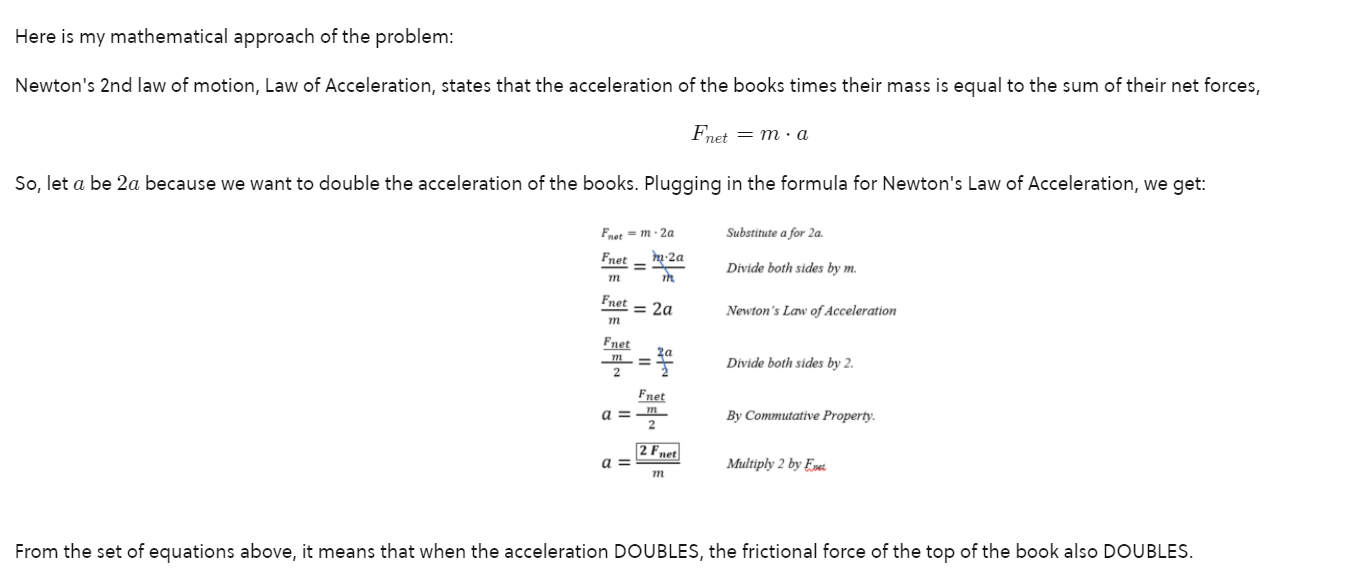# #2021.1: Solutions that I Got Correctly But Didn't Trust My Guts- Friction on Friction (3/11/2021)

Hello everyone. I am creating a SERIES of note walls for my profile, where every solution I did to problems in Brilliant were correctly done but mismanaged to get the right answer. In other words, I DID NOT TRUST MY GUTS AND I F&@KED UP!!! (Sorry about the language.) Because of this, I am not be able to post my solutions to the problem and I can no longer share my approach but otherwise, do them here.

On this first episode, I want to share my solution to 3/11/2021 Daily Challenge—Friction on Friction.

So, to sum up the question from the daily challenge, what happens to the frictional force of the top book that is piled upon another book when both of their accelerations are doubled?Here is my mathematical approach of the problem:

Newton's 2nd law of motion, Law of Acceleration, states that the acceleration of the books times their mass is equal to the sum of their net forces. So by plugging in the formula,

$F_{net}=m\cdot a$ we will derived at the following solution:From the set of equations above, it means that when the acceleration DOUBLES, the frictional force of the books also DOUBLES.

Here concludes one of those frustrating days that "Oh! It's so close yet so far!" moments. This alleviates my frustration and hoping to keep my sanity together (perhaps, I'm overexaggerating on this). Until then, hoping Brilliant.org will allow users who got the wrong answer still post their solutions on the comments section of the problems. Until then... John, signing off! :"D

PS: Please feel free to give a critical feedback regarding my explanation in the comments section below. Whether it be correcting some lacking to my solution/explanation, elaborating more the concepts behind the problem that I solved, or any other else, it will be really beneficial to learn from you guys. I'm willing to hear it from you so I can further improve my explanation skills. Thank you. :"D

PS 2: I thank @Jason Gomez for checking out my mistake on the previous solution. Your valuable response make me understand more about the derivation of formulas like this. If you are curious to the discussions we made in the comments section below, I will post a history note attachment to make all of you, readers, have a sense on what is going on. I highly suggest you read our discussions.

Historical Note 2021.1.1: Previous faulty solution:

You can click on the image to zoom in better. Thanks. ;)Note by John Bryan Galiza
4 months, 2 weeks ago

This discussion board is a place to discuss our Daily Challenges and the math and science related to those challenges. Explanations are more than just a solution — they should explain the steps and thinking strategies that you used to obtain the solution. Comments should further the discussion of math and science.

When posting on Brilliant:

• Use the emojis to react to an explanation, whether you're congratulating a job well done , or just really confused .
• Ask specific questions about the challenge or the steps in somebody's explanation. Well-posed questions can add a lot to the discussion, but posting "I don't understand!" doesn't help anyone.
• Try to contribute something new to the discussion, whether it is an extension, generalization or other idea related to the challenge.

MarkdownAppears as
*italics* or _italics_ italics
**bold** or __bold__ bold
- bulleted- list
• bulleted
• list
1. numbered2. list
1. numbered
2. list
Note: you must add a full line of space before and after lists for them to show up correctly
paragraph 1paragraph 2

paragraph 1

paragraph 2

[example link](https://brilliant.org)example link
> This is a quote
This is a quote
    # I indented these lines
# 4 spaces, and now they show
# up as a code block.

print "hello world"
# I indented these lines
# 4 spaces, and now they show
# up as a code block.

print "hello world"
MathAppears as
Remember to wrap math in $$ ... $$ or $ ... $ to ensure proper formatting.
2 \times 3 $2 \times 3$
2^{34} $2^{34}$
a_{i-1} $a_{i-1}$
\frac{2}{3} $\frac{2}{3}$
\sqrt{2} $\sqrt{2}$
\sum_{i=1}^3 $\sum_{i=1}^3$
\sin \theta $\sin \theta$
\boxed{123} $\boxed{123}$

Sort by:

You may write your solution down below a top solution or second top solution if you have got it wrong and feel that your solution has a different ideology compared to other solutions, also

$a=\dfrac{\frac{F_{net}}{m}}{2} = \frac{F_{net}}{2m} ≠ \frac{2F_{net}}{m}$

- 4 months, 2 weeks ago

Thank you for the advise regarding posting solutions if I got the answer wrong.

Also, thank you for noticing my mistake in the complex fraction part. Now that you pointed this out, it would now mean that for this solution, if you DOUBLE the mass of the object, you DOUBLE the acceleration, wouldn't it? I do not get then now if how am I supposed to bring a $2$ on the frictional force part of the equation. Can you please help me understand this so that I can re-fix my solution? I don't want to mislead anyone who will stop-by with the current information posted. Much appreciated from you. :)

- 4 months, 2 weeks ago

Oh, by the way. I managed to correct my solution by deriving a new one, which is the new one above. Is that how you derive the $2 F_{net}$ thingy?

- 4 months, 2 weeks ago

Yes it’s right now :) it can have been done easily by taking $F_{net}=ma$ therefore $F_{net} ∝ a$ which directly gives the answer

- 4 months, 2 weeks ago

Ah yes. I could not agree more to that. But I intended to do the step-by-step method so that I could also understand how the solution was derived at the first place. Anyways, thank you for taking your time to look upon my solution. Best regards. :)

- 4 months, 2 weeks ago